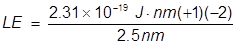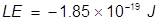# Lattice Energy Formula

Lattice Energy Formula

Lattice energy refers to the energy that is released when two oppositely charged gaseous ions attract to each other and form an ionic solid. The attraction of the two ions releases energy and the process is exothermic. Lattice energy can be a very complicated process but is often simplified by using Coulomb’s law.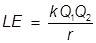LE = lattice energy

k =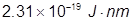Q1 and Q2 = numerical ion charges

r = the distance between the ion centers

Lattice Energy Formula Questions:

1. What is the lattice energy of a sodium ion and chlorine ion separated by 1.0 nm?

Note: nm refers to a nanometer or 10-9 m, sodium forms a +1 ion and chlorine forms a -1 ion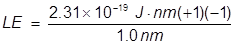2. What is the lattice energy of a sodium ion and oxide ion separated by 2.5 nm?